The QUANTREG Procedure

Confidence Interval

Subsections:

The QUANTREG procedure provides three methods to compute confidence intervals for the regression quantile parameter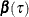: sparsity, rank, and resampling. The sparsity method is the most direct and the fastest, but it involves estimation of the sparsity function, which is not robust for data that are not independently and identically distributed. To deal with this problem, the QUANTREG procedure uses a local estimate of the sparsity function to compute a Huber sandwich estimate. The rank method, which computes confidence intervals by inverting the rank score test, does not suffer from this problem. However, the rank method uses the simplex algorithm and is computationally expensive with large data sets. The resampling method, which uses the bootstrap approach, addresses these problems, but at a computation cost.

Based on these properties, the QUANTREG uses a combination of the resampling and rank methods as the default. For data sets that have more than either 5,000 observations or more than 20 variables, the QUANTREG procedure uses the MCMB resampling method; otherwise it uses the rank method. You can request a particular method by using the CI= option in the PROC QUANTREG statement.

Sparsity

Consider the linear model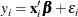Assume that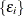,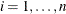, are iid with a distribution F and a density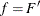, where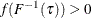in a neighborhood of. Under some mild conditions,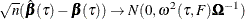where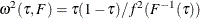and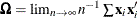(Koenker and Bassett 1982b).

This asymptotic distribution for the regression quantile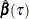can be used to construct confidence intervals. However, the reciprocal of the density function,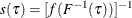which is called the sparsity function, must first be estimated.

Because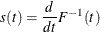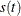can be estimated by the difference quotient of the empirical quantile function—that is,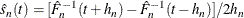whereis an estimate of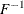and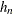is a bandwidth that tends to 0 as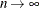.

The QUANTREG procedure provides two bandwidth methods. The Bofinger bandwidth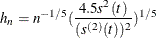is an optimizer of mean squared error for standard density estimation. The Hall-Sheather bandwidth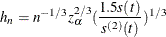is based on Edgeworth expansions for studentized quantiles, where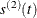is the second derivative ofand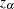satisfies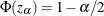for the construction of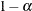confidence intervals. The following quantity is not sensitive to f and can be estimated by assuming f is Gaussian: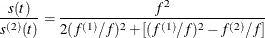can be estimated in either of the following ways:

• by the empirical quantile function of the residuals from the quantile regression fit,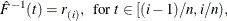• by the empirical quantile function of regression proposed by Bassett and Koenker (1982),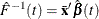The QUANTREG procedure interpolates the first empirical quantile function and produces the piecewise linear version: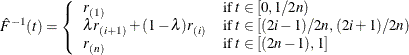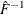is set to a constant if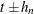falls outside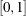.

This estimator of the sparsity function is sensitive to the iid assumption. Alternately, Koenker and Machado (1999) consider the non-iid case. By assuming local linearity of the conditional quantile function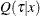in x, they propose a local estimator of the density function by using the difference quotient. A Huber sandwich estimate of the covariance and standard error is computed and used to construct the confidence intervals. One difficulty with this method is the selection of the bandwidth when using the difference quotient. With a small sample size, either the Bofinger or the Hall-Sheather bandwidth tends to be too large to assure local linearity of the conditional quantile function. The QUANTREG procedure uses a heuristic bandwidth selection in these cases.

By default, the QUANTREG procedure computes non-iid confidence intervals. You can request iid confidence intervals by specifying the IID option in the PROC QUANTREG statement.

Inversion of Rank Tests

The classical theory of rank tests can be extended to test the hypothesis: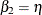in the linear regression model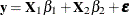. Here,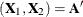. See Gutenbrunner and Jureckova (1992) for more details. By inverting this test, confidence intervals can be computed for the regression quantiles that correspond to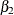.

The rank score function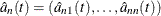can be obtained by solving the dual problem: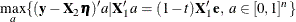For a fixed quantile, integrating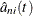with respect to the-quantile score function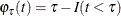yields the-quantile scores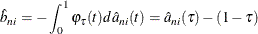Under the null hypothesis:,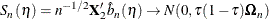for large n, where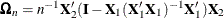.

Let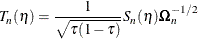Then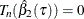from the constraint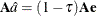in the full model. In order to obtain confidence intervals for, a critical value can be specified for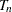. The dual vector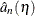is a piecewise constant in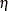, andcan be altered without compromising the optimality ofas long as the signs of the residuals in the primal quantile regression problem do not change. Whengets to such a boundary, the solution does change. But it can be restored by taking one simplex pivot. The process can continue in this way until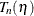exceeds the specified critical value. Becauseis piecewise constant, interpolation can be used to obtain the desired level of confidence interval (Koenker and d’Orey 1994).

Resampling

The bootstrap can be implemented to compute confidence intervals for regression quantile estimates. As in other regression applications, both the residual bootstrap and the xy-pair bootstrap can be used. The former assumes iid random errors and resamples from the residuals, whereas the latter resamples xy pairs and accommodates some forms of heteroscedasticity. Koenker (1994) considered a more interesting resampling mechanism, resampling directly from the full regression quantile process, which he called the Heqf bootstrap.

In contrast with these bootstrap methods, Parzen, Wei, and Ying (1994) observed that the following estimating equation for theregression quantile is a pivotal quantity for thequantile regression parameter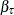: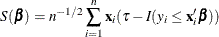In other words, the distribution of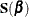can be generated exactly by a random vector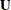, which is a weighted sum of independent, re-centered Bernoulli variables. They further showed that for large n, the distribution of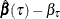can be approximated by the conditional distribution of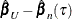, where
solves an augmented quantile regression problem by using n + 1 observations that have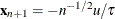and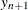sufficiently large for a given realization of u. By exploiting the asymptotically pivotal role of the quantile regression "gradient condition," this approach also achieves some robustness to certain heteroscedasticity.

Although the bootstrap method by Parzen, Wei, and Ying (1994) is much simpler, it is too time-consuming for relatively large data sets, especially for high-dimensional data sets. The QUANTREG procedure implements a new, general resampling method developed by He and Hu (2002), which is called the Markov chain marginal bootstrap (MCMB). For quantile regression, the MCMB method has the advantage that it solves p one-dimensional equations instead of solving p-dimensional equations, as the previous bootstrap methods do. This greatly improves the feasibility of the resampling method in computing confidence intervals for regression quantiles.